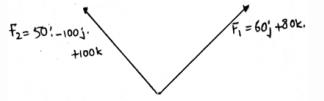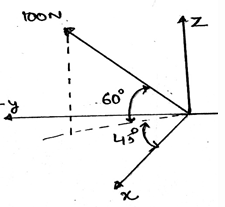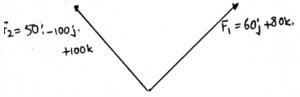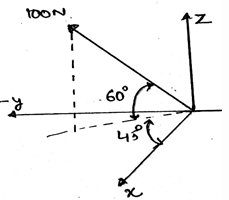Courses

# Test: Cartesian Vectors

## 30 Questions MCQ Test Engineering Mechanics | Test: Cartesian Vectors

Description
This mock test of Test: Cartesian Vectors for Mechanical Engineering helps you for every Mechanical Engineering entrance exam. This contains 30 Multiple Choice Questions for Mechanical Engineering Test: Cartesian Vectors (mcq) to study with solutions a complete question bank. The solved questions answers in this Test: Cartesian Vectors quiz give you a good mix of easy questions and tough questions. Mechanical Engineering students definitely take this Test: Cartesian Vectors exercise for a better result in the exam. You can find other Test: Cartesian Vectors extra questions, long questions & short questions for Mechanical Engineering on EduRev as well by searching above.
QUESTION: 1

### In right handed coordinate system which axis is considered to be positive?

Solution:

As right handed coordinate system means that you are curling your fingers from positive x-axis towards y-axis and the thumb which is projected is pointed to the positive z-axis. Thus visualizing the same and knowing the basic members of axis will not create much problem.

QUESTION: 2

### If A is any vector with Ai + Bj + Ck then what is the y-axis component of the vector?

Solution:

As the given vector is shown in the Cartesian system, the number with “j” is the Y-axis component of the given vector. With the numbers with “i” representing components of x-axis and “k” representing the z axis respectively.

QUESTION: 3

### If the force vector F is having its x-axis component being equal to Z N, y-axis component be X N and z-axis component be Y N then vector F is best represented by?

Solution:

It is given that x, y and z-axis components are Z, X and Y respectively. Thus, just by placing the right coordinates we get option d to be correct. Here just the interchange of the axis’s representor with their respective axis is creating confusion. Which must be figured out.

QUESTION: 4

Which statement is right for force vector F = Ai + Bj + Ck?

Solution:

As given the vector is F = Ai + Bj + Ck, this implies that the x ,y and z-axis components of this vector is A, B and C respectively. But, in rectangular components representation of any vector, the vector is written as F = Fx + Fy + Fz.

QUESTION: 5

What is the magnitude of the Cartesian vector having the x, y and z axis components to be A, B and C?

Solution:

The magnitude of a Cartesian vector having the x, y and z axis components to be A, B and C is always the square root of the squares each A, B and C. This comes from the distance formula between two points in the Cartesian plane. That is the square root of the subtraction of final and initial point of line.

QUESTION: 6

What is cosα for force vector F = Ax + By +Cz (Given α, β and γ are the angles made by the vector with x, y and z axis respectively) ?

Solution:

The cosine component of the vector is defined as the ratio of the x-axis component to the magnitude of the vector, i.e. F in this case. Likewise the sine component is the ratio of y-axis component to the magnitude of the vector.

QUESTION: 7

What is the sum of squares of the cosine angles made by the force vector with the coordinate axis?

Solution:

The sum of the squares of the cosines of the vector will give you the squares of the components in the numerator, and the vector’s magnitude’s square in the denominator. But the numerator sum is equal to the vector’s magnitude’s square. Thus, the answer = 1.

QUESTION: 8

What is x-axis component of the force vector Ai + Bj +Ck with magnitude equal to F?

Solution:

As we know that the cosα is the ratio of the x-axis component to the magnitude of the vector. Thus the x-axis components is Fcosα, F, the magnitude in the case. Likely if we want to take the y-axis component we would try to do the same with the sine component.

QUESTION: 9

We can add the force vectors directly. But with dividing each by it’s magnitude first. (True/False)

Solution:

False, because if you will divide the magnitude of the vector to itself than the resulting would be the unit vector. Which is just giving you the direction of the vector, not the vector itself. This means unit vector has direction same as it’s respective vector but having magnitude equal to one.

QUESTION: 10

For a vector F, Fcosβ is equal to zero. What does this refer?

Solution:

As we know the α, β and γ are the angles made by the x, y and z-axis respectively. Thus y-axis component is zero, or β = 90˚. And thus if the angle is giving component to be zero this means the vector in that particular axis is perpendicular to that axis.

QUESTION: 11

Which statement is correct about the vector F?

Solution:

As we know the α, β and γ are the angles made by the x, y and z-axis respectively. Thus, is the magnitude of the vector is F, the F= Fcos β + Fcos α + Fcosγ. Which means the force is the resultant of all its axis’ components.

QUESTION: 12

Which is true?

Solution:

The total of two or more forces is equal to the sum of their respective axis’s components. That is the resultant adds up all the components of the forces in their respective axis, whether it may be x, y or z axis.

QUESTION: 13

Find the angle α, for the vector making an angle by y and z axis as 60˚ and 45˚ respectively. It makes an angle of α with x-axis. The magnitude of the force is 200N.

Solution:

When you will resolve the vector in its x, y and z-axis components, you will get an equation containing cosα. After getting the α correctly, you need to directly put that value in the previous equation of components. α = 60˚. As 120˚ will give negative component. Just try to resolve the vector in its components.

QUESTION: 14

What is the magnitude of the resultant force when F1 = 60j + 80k and F2 = 50i – 100j + 100k?Solution:

Just do the vector addition. And then calculate the resultant of the sum you get. In vector addition just use the x, y and z axis components and then add them separately which will give you the resultant’s components. Now do the pythogerous method of square rooting of components and the resultant is what you get.

QUESTION: 15

Express F = 100 N (shown in the figure below) as a Cartesian vector.Solution:

Try to resolve the given vector so that you will get respective components. Use sine and cosine multiplication of the angles given to calculate the components. Also try to use the angles given in the diagram which will be easier way to solve the question and getting the answer.

QUESTION: 16

Express the vector in the Cartesian Form, if the angle made by it with y and z axis is 60˚ and 45˚ respectively. Also it make an angle of α with x-axis. The magnitude of the force is 200N.

Solution:

When you will resolve the vector in its x, y and z-axis components, you will get an equation containing cosα. After getting the correctly, you need to directly put that value in the previous equation of components.

QUESTION: 17

In the figure given below, with F1 = 60j + 80k and F2= 50i -100j + 100k, what is the value of β? (β is the angle made by the resultant vector with the y-axis)Solution:

Just add the two vectors in their Cartesian form only. After that you will get the resultant vector in the Cartesian form. After that just divide the y-axis component’s magnitude by the resultant of the vector. You will get cosβ.

QUESTION: 18

In the figure given below, calculate the value of cosγ. (γ is the angle made by the resultant vector and the z-axis)Solution:

Well, the question is just a tricky one. Just subtract 60 from 90, you will get the γ, which is 30. And then apply the cosine formula for getting the components of respective axis.

QUESTION: 19

What is the magnitude of the vector, 12i – 8j – 24k?

Solution:

122 + 82 + 242 = 282 . After you get this, the 28 is the magnitude. This is the using of distance formula between two Cartesian points. Considering the point (12, -8, -24) and then calculating the distance from the origin.

QUESTION: 20

The coordinate of the Force vector AB is A (2, 0, 2) and B (-2, 3.46, 3). What are its directions?

Solution:

First subtract the coordinates of the A form B. And place the x, y and z-axis coordinate separated by i, j and k respectively. Like Ai + Bj + Ck , if A, B and C are the x, y and z-axis coordinates. You will get a Cartesian vector, just find its magnitude and divide it with the vector.

QUESTION: 21

The coordinate of the Force vector AB is A (2, 0, 2) and B (-2, 3.46, 3). It has a magnitude of 750N. Which is the best Cartesian representation of the vector AB?

Solution:

First try to make the unit vector of AB. Then you will get the Cartesian form of unit vector, after that just multiply 750 scalar quantity with it. Which means you are first taking out the desired direction and then multiplying the scalar to get the desired vector in desired direction.

QUESTION: 22

A force vector is along 4i – 4k direction and has a magnitude 100N and another force vector is along 4i +2j -4k and has a magnitude of 120N. What is the resultant of both forces?

Solution:

Just make the unit vectors of the given directions of the vectors and then multiply them with their respective magnitudes, after than you will get two vectors in the Cartesian form. Just add them.

QUESTION: 23

The value cos-1(-3/7) + cos-1(2/7) + cos-1(6/7) is ____________

Solution:

It’s a calculative question, but still can be solved if you construct the triangle of the respective cosine inverses given, then convert them in the tangent form then use log table, if necessary. Or use of calculator is also good if permissible. Or you can make use of triangle laws to obtain the same.

QUESTION: 24

Two vectors emerging from a point are always in a single plane.

Solution:

Yes, whenever two vectors are there, they are always in a single plane. If the emerging vectors are more than three then saying about their plane is somewhat difficult. But for two vectors it seems easy to understand about their plane.

QUESTION: 25

Three vectors emerging from a point are always in a single plane.

Solution:

Yes, whenever two vectors are there, they are always in a single plane. But you cannot say about the three vectors, they may or may be. Because if the emerging vectors are more than three then saying about their plane is somewhat difficult. But for two vectors it seems easy to understand about their plane.

QUESTION: 26

What is the magnitude of unit vector?

Solution:

The magnitude of the unit vector is always = 1. This means unit vector is the vector in the direction of the original vector and the magnitude of the same is unity. And unit vector is always in the direction of its vector.

QUESTION: 27

What is the difference between position vector and unit vector?

Solution:

As position vector defines the position of the point in the Cartesian plane wit respect of the origin, it will definitely have a direction and also a magnitude. Unit vector is a vector whose magnitude is = 1 and is having a specific direction.

QUESTION: 28

What if we multiply a scalar to the unit vector?

Solution:

The magnitude of the unit vector will change by the amount of the scalar multiplied. Not the direction. Because the direction is being fixed after the application of the unit vector’s calculation. That is the vector divided by its magnitude.

QUESTION: 29

Which is true for the vector provided only position coordinates given?

Solution:

We find the vector form from subtracting the initial position coordinates from final position coordinates. Not from subtracting the final position coordinates from initial position coordinates.

QUESTION: 30

A vector can always have_____________

Solution:

All vectors have three components, whether they are zero or not. And unit vector is always in the direction of its vector. And the unit vector has the magnitude equal to unity. This means unit vector is the vector in the direction of the original vector and the magnitude of the same is unity.